# Fixed-point property

This article defines a property of topological spaces: a property that can be evaluated to true/false for any topological space|View a complete list of properties of topological spaces

## Definition

A topological space is said to have the fixed-point property if every continuous map (not necessarily a self-homeomorphism) from the topological space to itself has a fixed point.

## Examples

### Manifolds without boundary

Manifold or family of manifolds Dimension in terms of parameter Does it satisfy the fixed-point property? Proof/explanation
sphere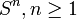$S^n,n \ge 1$$n$ No The antipodal map is a fixed-point-free self-map (in fact, it's a self-homeomorphism without fixed points).
real projective space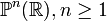$\mathbb{P}^n(\R), n \ge 1$$n$ Even$n$: Yes
Odd$n$: No
See real projective space has fixed-point property iff it has even dimension
complex projective space$\mathbb{P}^n(\mathbb{C}), n \ge 1$$2n$ Even$n$: Yes
Odd$n$: No
See complex projective space has fixed-point property iff it has even complex dimension.
quaternionic projective space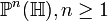$\mathbb{P}^n(\mathbb{H}), n \ge 1$$4n$ Even$n$: Yes
Odd$n$: No
See quaternionic projective space has fixed-point property iff it has even quaternionic dimension.
compact orientable surface of genus$g \ge 0$ 2 No
torus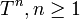$T^n, n \ge 1$, product of$n$ circles$n$ No Follows from the fact that it's a nontrivial Lie group, so multiplication by a non-identity element. Also, we can use the coordinate-wise antipodal map.
product of spheres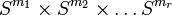$S^{m_1} \times S^{m_2} \times \dots S^{m_r}$$m_1 + m_2 + \dots + m_r$ No Take the antipodal map for each coordinate sphere.

## Facts

### Fixed-point theorems

In general, we combine the Lefschetz fixed-point theorem with the structure of the cohomology ring of the space to determine whether or not it has the fixed-point property. For instance, we can show that complex projective space in even dimensions has the fixed-point property, by combining the Lefschetz fixed-point theorem with the fact that the trace on the$(2k)^{th}$ homology is$d^k$ where$d$ is the trace on the second homology.

### Products

The product of any topological space that does not satisfy the fixed-point property with any nonempty topological space gives a space that does not satisfy the fixed-point property.

## Metaproperties

### Retract-hereditariness

This property of topological spaces is hereditary on retracts, viz if a space has the property, so does any retract of it
View all retract-hereditary properties of topological spaces

Every retract of a space with the fixed-point property also has the fixed-point property. Further information: fixed-point property is retract-hereditary